# 8 1 Solving Quadratic Equations Many quadratic equations

• Slides: 24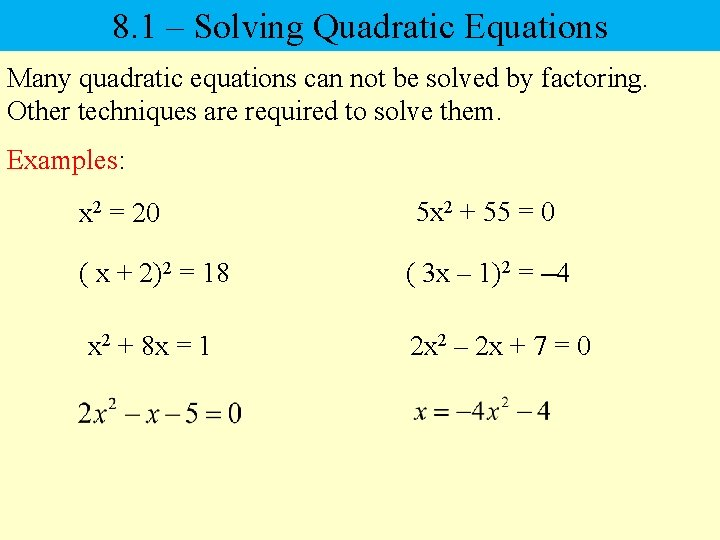8. 1 – Solving Quadratic Equations Many quadratic equations can not be solved by factoring. Other techniques are required to solve them. Examples: x 2 = 20 5 x 2 + 55 = 0 ( x + 2)2 = 18 ( 3 x – 1)2 = – 4 x 2 + 8 x = 1 2 x 2 – 2 x + 7 = 08. 1 – Solving Quadratic Equations Square Root Property If b is a real number and if a 2 = b, then a = ±√¯‾. b x 2 = 20 5 x 2 + 55 = 0 x = ±√‾‾ 20 5 x 2 = – 55 x = ±√‾‾‾‾ 4· 5 x 2 = – 11 x = ± 2√‾ 5 – 11 x = ±√‾‾‾ x = ± i√‾‾‾ 11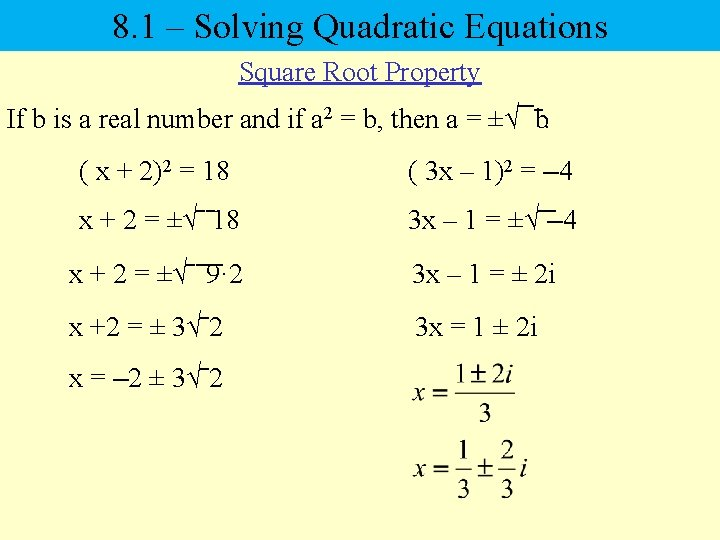8. 1 – Solving Quadratic Equations Square Root Property If b is a real number and if a 2 = b, then a = ±√¯‾. b ( x + 2)2 = 18 ( 3 x – 1)2 = – 4 x + 2 = ±√‾‾ 18 3 x – 1 = ±√‾‾– 4 x + 2 = ±√‾‾‾‾ 9· 2 3 x – 1 = ± 2 i x +2 = ± 3√‾ 2 3 x = 1 ± 2 i x = – 2 ± 3√‾ 2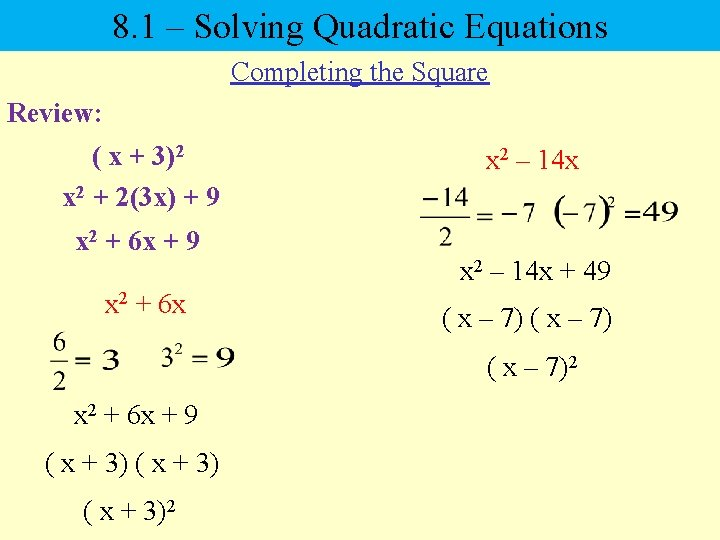8. 1 – Solving Quadratic Equations Completing the Square Review: ( x + 3)2 x 2 + 2(3 x) + 9 x 2 + 6 x x 2 – 14 x + 49 ( x – 7)2 x 2 + 6 x + 9 ( x + 3)2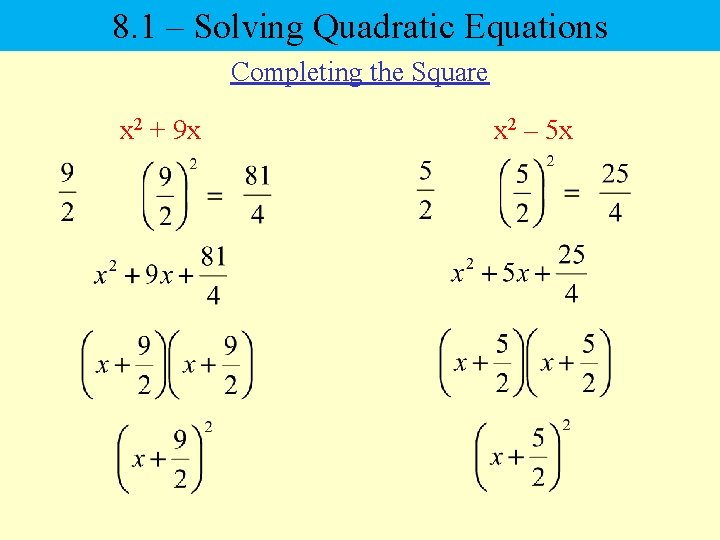8. 1 – Solving Quadratic Equations Completing the Square x 2 + 9 x x 2 – 5 x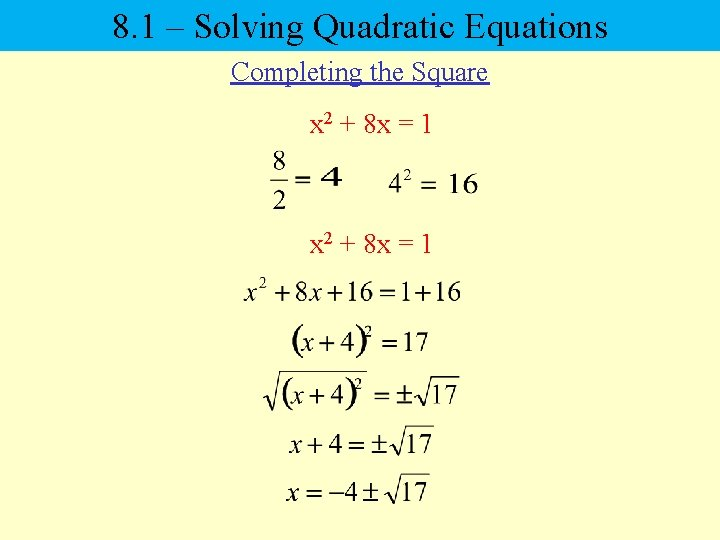8. 1 – Solving Quadratic Equations Completing the Square x 2 + 8 x = 1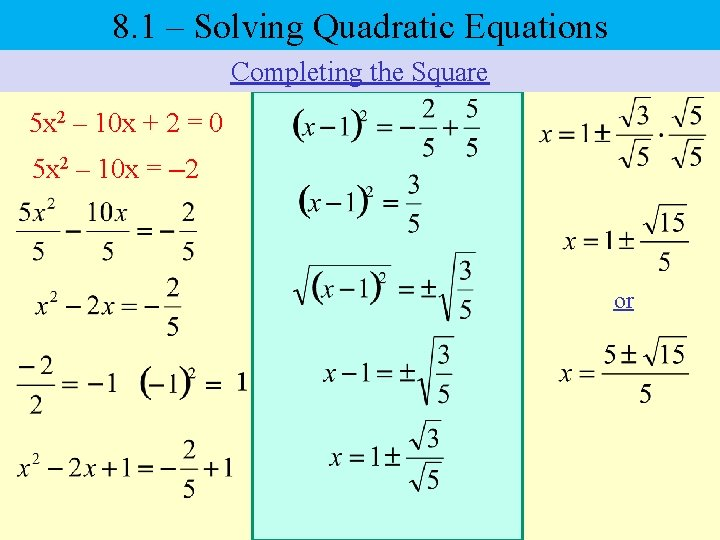8. 1 – Solving Quadratic Equations Completing the Square 5 x 2 – 10 x + 2 = 0 5 x 2 – 10 x = – 2 or8. 1 – Solving Quadratic Equations Completing the Square 2 x 2 – 2 x + 7 = 0 2 x 2 – 2 x = – 7 or8. 2 – Solving Quadratic Equations The Quadratic Formula The quadratic formula is used to solve any quadratic equation. Standard form of a quadratic equation is: The quadratic formula is:8. 2 – Solving Quadratic Equations The Quadratic Formula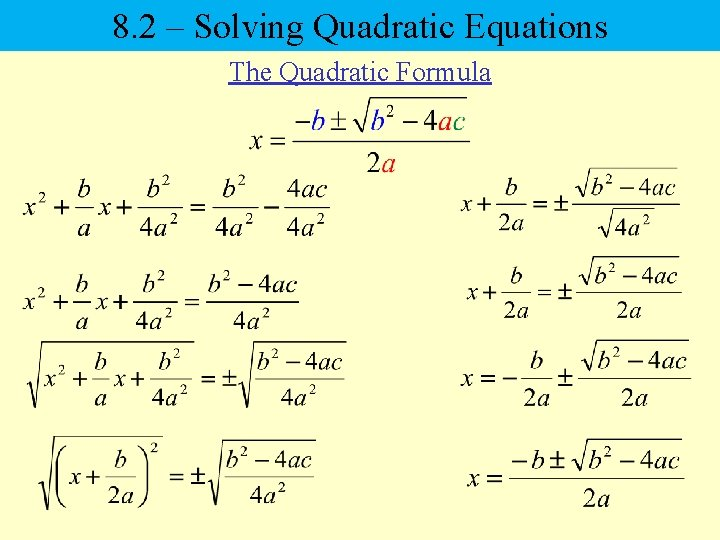8. 2 – Solving Quadratic Equations The Quadratic Formula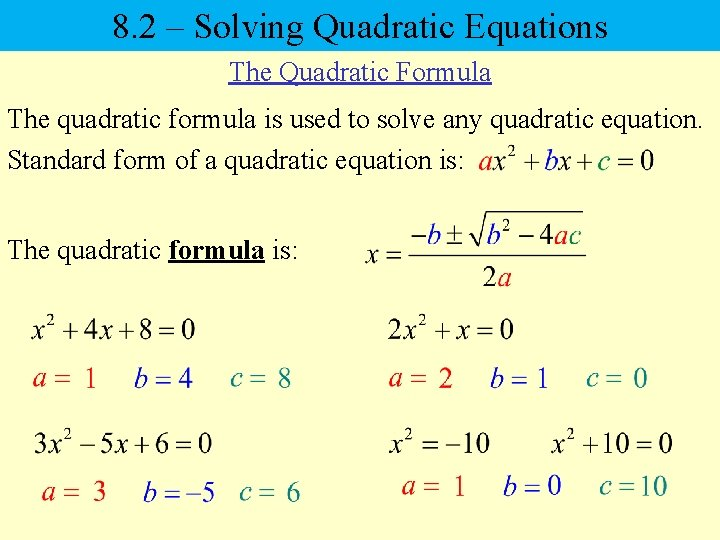8. 2 – Solving Quadratic Equations The Quadratic Formula The quadratic formula is used to solve any quadratic equation. Standard form of a quadratic equation is: The quadratic formula is: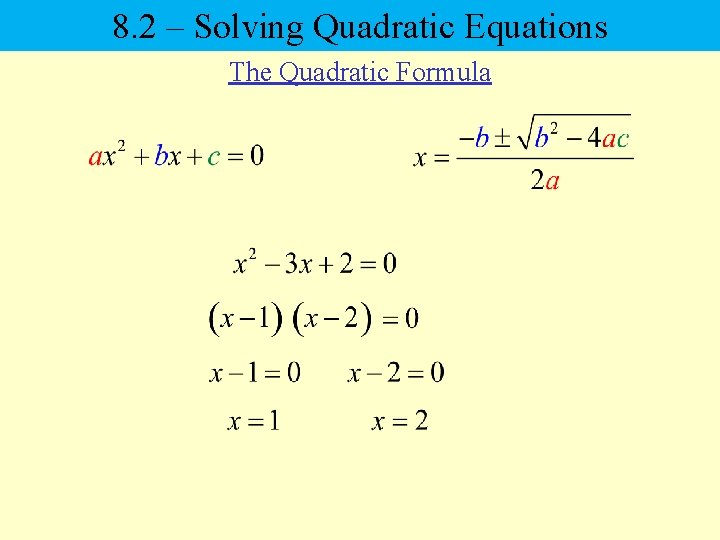8. 2 – Solving Quadratic Equations The Quadratic Formula8. 2 – Solving Quadratic Equations The Quadratic Formula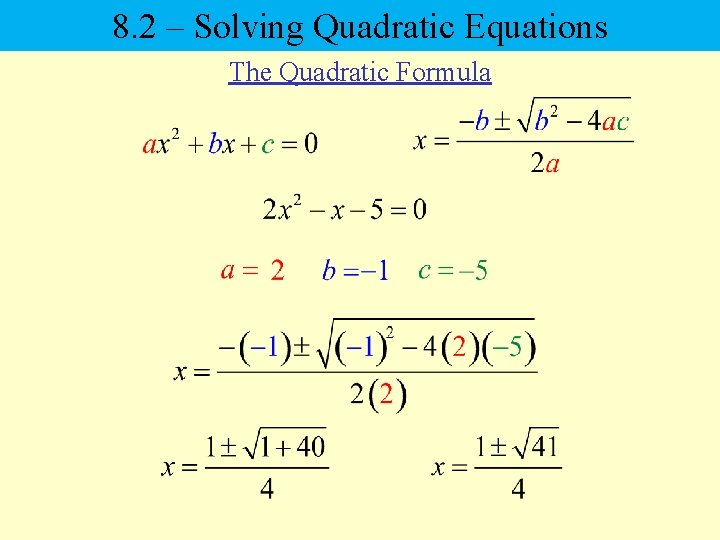8. 2 – Solving Quadratic Equations The Quadratic Formula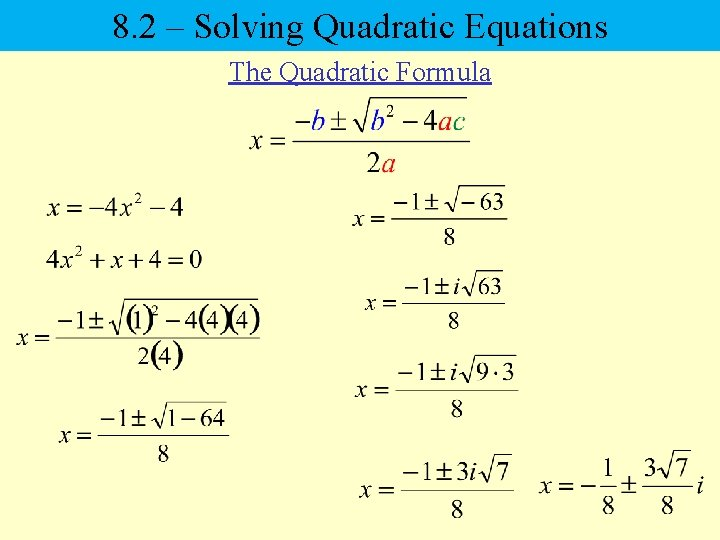8. 2 – Solving Quadratic Equations The Quadratic Formula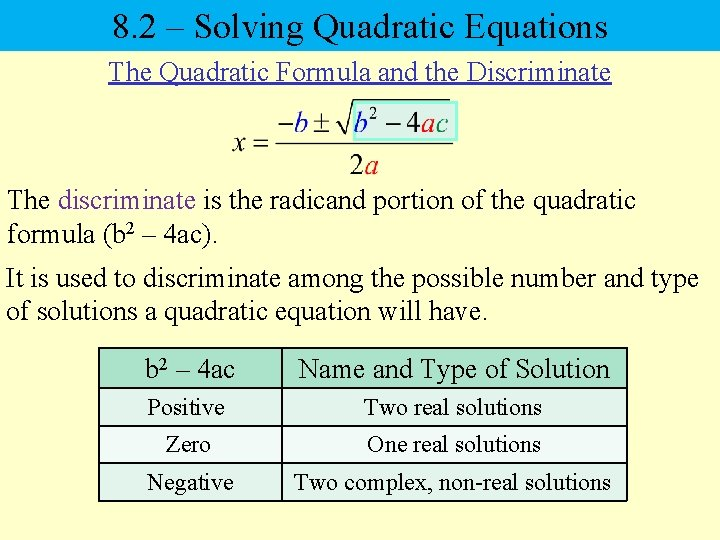8. 2 – Solving Quadratic Equations The Quadratic Formula and the Discriminate The discriminate is the radicand portion of the quadratic formula (b 2 – 4 ac). It is used to discriminate among the possible number and type of solutions a quadratic equation will have. b 2 – 4 ac Name and Type of Solution Positive Two real solutions Zero One real solutions Negative Two complex, non-real solutions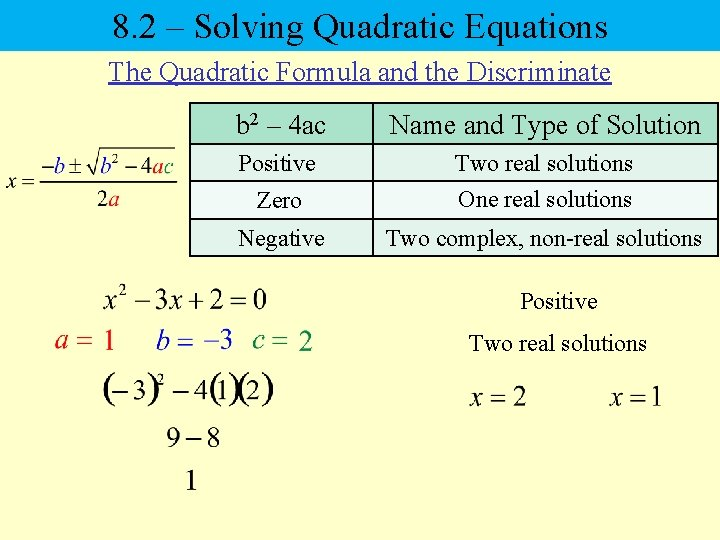8. 2 – Solving Quadratic Equations The Quadratic Formula and the Discriminate b 2 – 4 ac Name and Type of Solution Positive Two real solutions Zero One real solutions Negative Two complex, non-real solutions Positive Two real solutions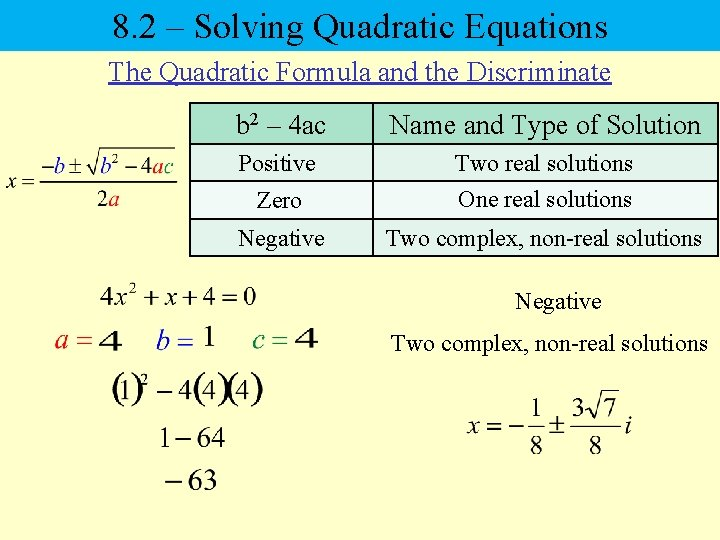8. 2 – Solving Quadratic Equations The Quadratic Formula and the Discriminate b 2 – 4 ac Name and Type of Solution Positive Two real solutions Zero One real solutions Negative Two complex, non-real solutions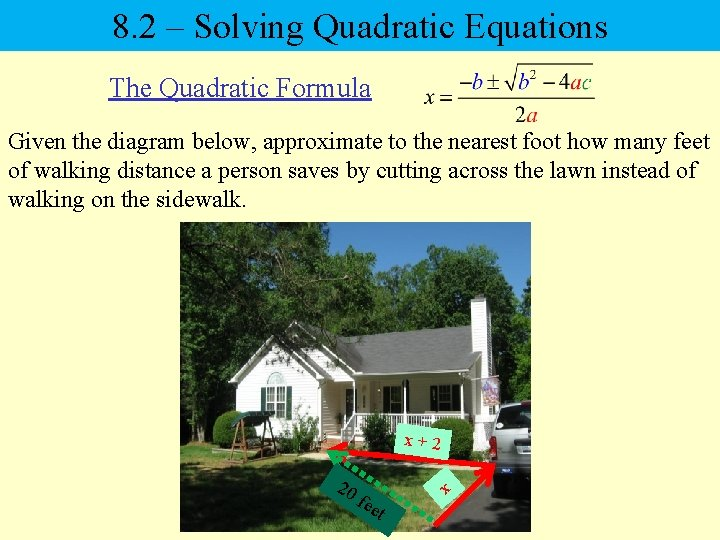8. 2 – Solving Quadratic Equations The Quadratic Formula Given the diagram below, approximate to the nearest foot how many feet of walking distance a person saves by cutting across the lawn instead of walking on the sidewalk. x+2 20 x fee t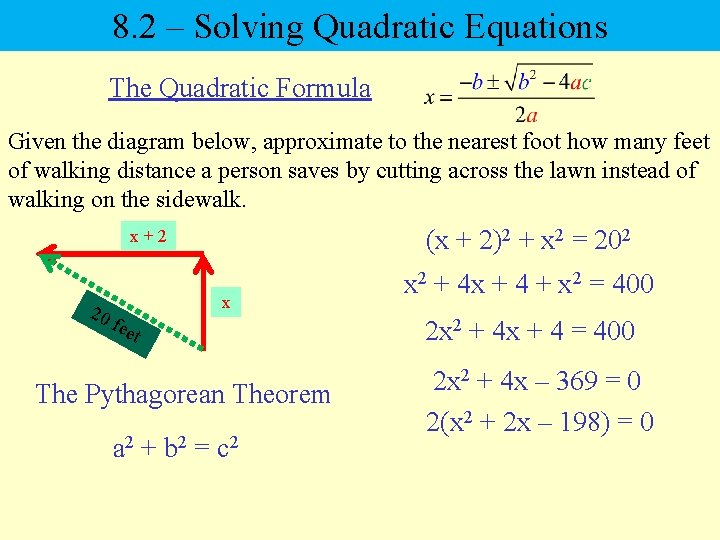8. 2 – Solving Quadratic Equations The Quadratic Formula Given the diagram below, approximate to the nearest foot how many feet of walking distance a person saves by cutting across the lawn instead of walking on the sidewalk. (x + 2)2 + x 2 = 202 x+2 20 fee t x The Pythagorean Theorem a 2 + b 2 = c 2 x 2 + 4 x + 4 + x 2 = 400 2 x 2 + 4 x + 4 = 400 2 x 2 + 4 x – 369 = 0 2(x 2 + 2 x – 198) = 0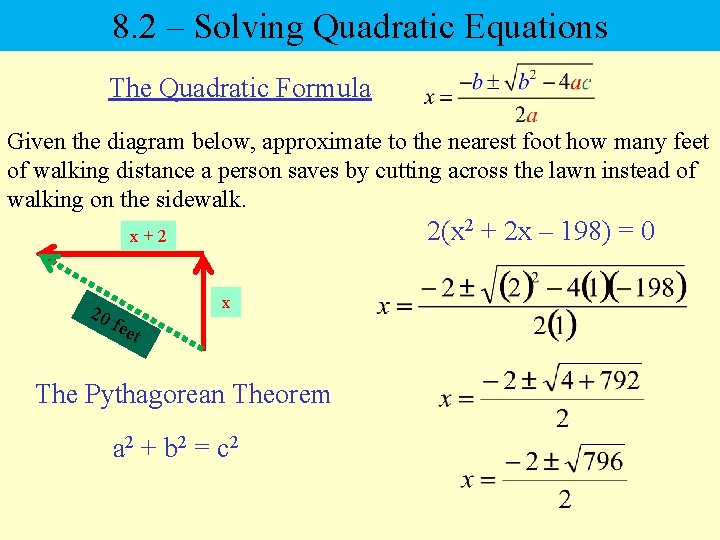8. 2 – Solving Quadratic Equations The Quadratic Formula Given the diagram below, approximate to the nearest foot how many feet of walking distance a person saves by cutting across the lawn instead of walking on the sidewalk. 2(x 2 + 2 x – 198) = 0 x+2 20 fee t x The Pythagorean Theorem a 2 + b 2 = c 2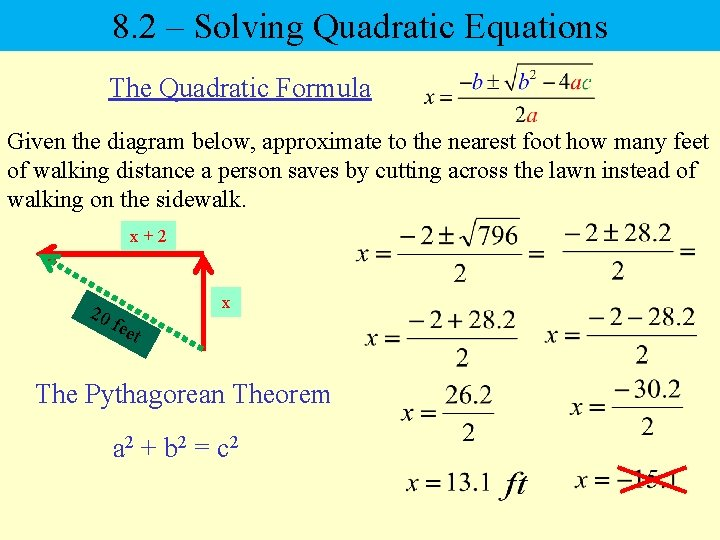8. 2 – Solving Quadratic Equations The Quadratic Formula Given the diagram below, approximate to the nearest foot how many feet of walking distance a person saves by cutting across the lawn instead of walking on the sidewalk. x+2 20 fee t x The Pythagorean Theorem a 2 + b 2 = c 28. 2 – Solving Quadratic Equations The Quadratic Formula Given the diagram below, approximate to the nearest foot how many feet of walking distance a person saves by cutting across the lawn instead of walking on the sidewalk. x+2 20 fee t x The Pythagorean Theorem a 2 + b 2 = c 2 28 – 20 = 8 ft